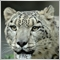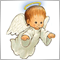# How to make bool in an array[]882

Hello everyone,

I'm not sure how to do this.  Sorry not very helpful code snippet, can it be achieved?

``` if(MRH<MO){c=true;}
if(MRH>MO){d=true;}
if(MRL<MO){e=true;}
if(MRL>MO){f=true;}
if(MRH>MRL){g=true;}
if(MRH<MRL){h=true;}
if(CR<OR){i=true;}
if(CR>OR){j=true;}
if(g==h){DlWI()};```3037

GrumpyDuckMan:

Hello everyone,

I'm not sure how to do this.  Sorry not very helpful code snippet, can it be achieved?

I'm sorry I really don't know what you want to achieve. I've been thinking about that for almost 5 minutes. But I have no idea. Creating a bool array? Triggering DlWI() when MRH==MRL? Your set of conditions is a bit nonsense.
BTW I usually set a bool variable this way c=MRH<MO; instead of if(MRH<MO){c=true;}
But it depends what you want to achieve.882

Petr Nosek:
I'm sorry I really don't know what you want to achieve. I've been thinking about that for almost 5 minutes. But I have no idea. Creating a bool array? Triggering DlWI() when MRH==MRL? Your set of conditions is a bit nonsense.
BTW I usually set a bool variable this way c=MRH<MO; instead of if(MRH<MO){c=true;}
But it depends what you want to achieve.

Hello again,

Something like this.

`bool= Conditions[state]`

where state identified bool Conditions[]

"BTW I usually set a bool variable this way c=MRH<MO; instead of if(MRH<MO){c=true;}"

MRH, MO aren't bool3037

GrumpyDuckMan:

"BTW I usually set a bool variable this way c=MRH<MO; instead of if(MRH<MO){c=true;}"

MRH, MO aren't bool

Of course MRH and MO needn't be bool. If MRH is less than MO then c is true otherwise c is false.3037

GrumpyDuckMan:

Hello again,

Something like this.

where state identified bool Conditions[]

I still don't know what you want to achieve.

I write an example how you can work with conditions.

```   bool arr;
double X1=1.0,X2=0.0,X3=2.0;
arr=X1<X2;  // In this case is false
arr=X1<X3;  // In this case is true
arr=X2<X3;  // In this case is true
int count=ArraySize(arr);
bool met=true;
// Check if all condition are met
for(int i=0;i<count;i++) met&=arr[i];
if(met) Print("All the condition are met");
else Print("Not all the condition are met");```882

Petr Nosek:

I still don't know what you want to achieve.

I write an example how you can work with conditions.

Hello again,

I believe that I may have solved the problem. Still a work in process atm.